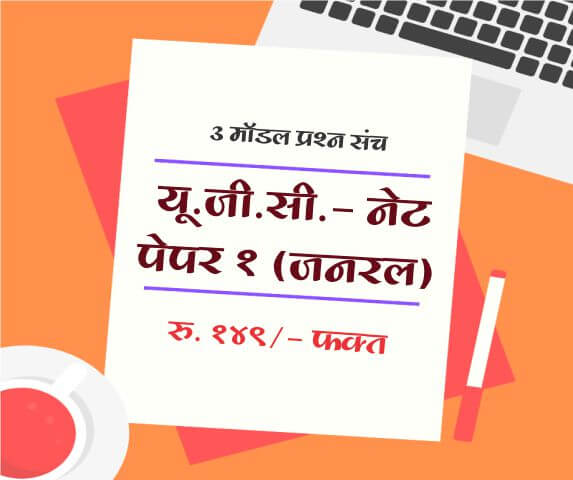# Hyperbolic Functions

In mathematics, hyperbolic functions are analogs of the ordinary trigonometric, or circular functions.

The basic hyperbolic functions are the hyperbolic sine "sinh" and the hyperbolic cosine "cosh" from which are derived the hyperbolic tangent "tanh" hyperbolic cosecant "csch" or "cosech" or hyperbolic secant "sech" and hyperbolic cotangent "coth" corresponding to the derived trigonometric functions.

Some formulas are as given below

1. $$Cosh^2 θ - Sinn^2 θ = 1$$
2. $$Sech^2 θ + tanh^2 θ = 1$$
3. $$Coth^2 θ - Cosech^2 θ = 1$$
4. $$Sinh (A + B) = Sinh A.Cosh B + Cosh A.Sinh B$$
5. $$Sinh (A - B) = Sinh A. Cosh B - Cosh A. Sinh B$$
6. $$Cosh (A - B) = Cosh A Cosh B + Sinh A Sinh B$$
7. $$Cosh (A - B) = Cosh A Cosh B - Sinh A Sinh B$$
8. $$tanh (A + B) = {tanh A - tanh B \over 1 - tanh A tanh B}.$$
9. $$tanh (A - B) = {tanh A - tanh B \over 1 + tanh A tanh B}.$$
10. $$sinh 2A = 2 sinh A . Cosh B$$
& $$sinh 2A = {2tanh A \over 1 - tanh^2 A}.$$
11. $$cosh 2A = cosh^2 A + sinh^2 A$$
$$cosh 2A = 1 + 2 sinh^2 A$$
$$cosh 2A = 2cosh^2 A - 1$$
$$cosh 2A = 2cosh^2 A - 1$$ $$cosh 2A = {1 + tanh^2 A \over 1 - tanh^2 A}.$$
OR
$$cosh 2A + 1 = 2 cosh^2 A$$
OR
$$cosh 2A - 1 = 2sinh^2 A$$
12. $$tanh 2A = {2 tanh A\over 1 + tanh^2 A}.$$
13. $$sinh 3A = 3 sinh A + 4 sinh^3 A$$
14. $$cosh 3A = 4cosh^3 A - 3cosh A$$
15. $$tanh 3A = {3tanh A + tanh^3 B\over 1 + 3tanh^2 A}.$$
16. $$sinh (A + B) + sinh (A - B) = 2sinh A cosh B$$
17. $$sinh (A + B) - sinh (A - B) = 2cosh A sinh B$$
18. $$cosh (A + B) + cosh (A - B) = 2cosh A cosh B$$
19. $$cosh (A + B) - cosh (A - B) = 2sinh A sinh B$$
20. $$sinh A + sinh B = 2sinh ({A + B \over 2}) cosh ({A - B \over 2})$$
21. $$sinh A - sinh B = 2cosh ({A + B \over 2}) sinh ({A - B \over 2})$$
22. $$cosh A + cosh B = 2cosh ({A + B \over 2}) cosh ({A - B \over 2})$$
23. $$cosh A - cosh B = 2sinh ({A + B \over 2}) sinh B ({A - B \over 2})$$

Labels: MPSC, TET, NET-SET, Maths, Mathamatics, Hyperbolic Functions

#### Articles

 Name : Comment :
Upcoming Exam Forms

Full Length Mock Tests
Timer Based Exams
Instant Result and assesment
Detailed analasys of Result##### UGC NET SET Paper 1 Mock Tests

UGC-NET / SET Paper 1 mock test

3 Mock Tests, 150 Questions with Explanations

Rs: 149 Only/-##### LAW MH-CET 2019 (3 YEAR COURSE)

Mock test for MH CET Law 2019 for three year law programme

3 Mock Tests, 450 Questions with Explanations

Rs: 249 Only/-##### यू. जी. सी. - नेट पेपर १ (जनरल)

यू. जी. सी. - नेट पेपर १ (अनिवार्य) सामान्य प्रश्न हिंदीमें

3 Mock Tests, 150 Questions with Explanations

Rs: 149 Only/-